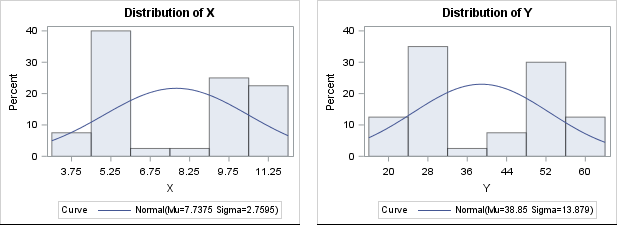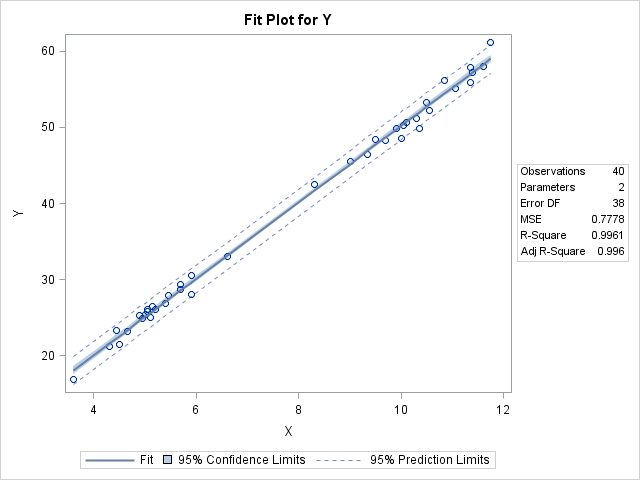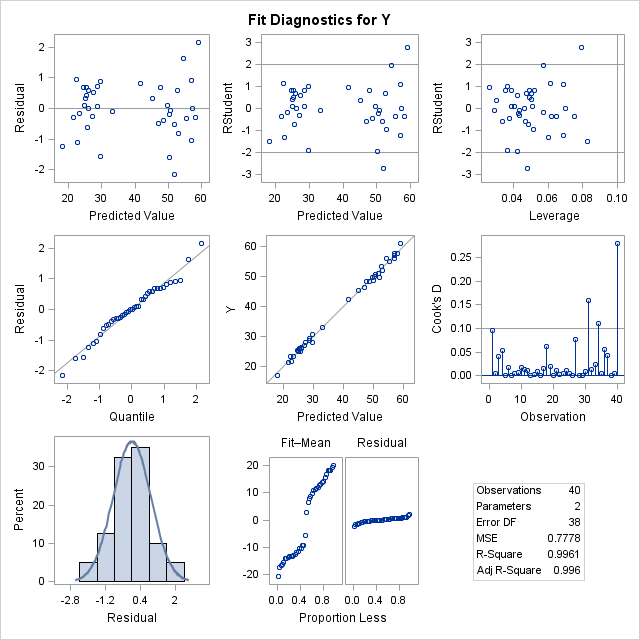A frequent topic on SAS discussion forums is how to check the assumptions of an ordinary least squares linear regression model. Some posts indicate misconceptions about the assumptions of linear regression. In particular, I see incorrect statements such as the following:

• Help! A histogram of my variables shows that they are not normal! I need to apply a normalizing transformation before I can run a regression....
• Before I run a linear regression, I need to test that my response variable is normal....

Let me be perfectly clear: The variables in a least squares regression model do not have to be normally distributed. I'm not sure where this misconception came from, but perhaps people are (mis)remembering an assumption about the errors in an ordinary least squares (OLS) regression model. If the errors are normally distributed, you can prove theorems about inferential statistics such as confidence intervals and hypothesis tests for the regression coefficients. However, the normality-of-errors assumption is not required for the validity of the parameter estimates in OLS. For the details, the Wikipedia article on ordinary least squares regression lists four required assumptions; the normality of errors is listed as an optional fifth assumption.

In practice, analysts often "check the assumptions" by running the regression and then examining diagnostic plots and statistics. Diagnostic plots help you to determine whether the data reveal any deviations from the assumptions for linear regression. Consequently, this article provides a "getting started" example that demonstrates the following:

1. The variables in a linear regression do not need to be normal for the regression to be valid.
2. You can use the diagnostic plots that are produced automatically by PROC REG in SAS to check whether the data seem to satisfy some of the linear regression assumptions.

By the way, don't feel too bad if you misremember some of the assumptions of linear regression. Williams, Grajales, and Kurkiewicz (2013) point out that even professional statisticians sometimes get confused. And if you want to be pedantic, checking the residuals after a model is run is not proof that the modeling assumptions are true!

### An example of nonnormal data in regression

Consider this thought experiment: Take any explanatory variable, X, and define Y = X. A linear regression model perfectly fits the data with zero error. The fit does not depend on the distribution of X or Y, which demonstrates that normality is not a requirement for linear regression.

For a numerical example, you can simulate data such that the explanatory variable is binary or is clustered close to two values. The following data shows an X variable that has 20 values near X=5 and 20 values near X=10. The response variable, Y, is approximately five times each X value. (This example is modified from an example in Williams, Grajales, and Kurkiewicz, 2013.) Neither variable is normally distributed, as shown by the output from PROC UNIVARIATE:

```/* For n=1..20, X ~ N(5, 1). For n=21..40, X ~ N(10, 1). Y = 5*X + e, where e ~ N(0,1) */ data Have; input X Y @@; datalines; 3.60 16.85 4.30 21.30 4.45 23.30 4.50 21.50 4.65 23.20 4.90 25.30 4.95 24.95 5.00 25.45 5.05 25.80 5.05 26.05 5.10 25.00 5.15 26.45 5.20 26.10 5.40 26.85 5.45 27.90 5.70 28.70 5.70 29.35 5.90 28.05 5.90 30.50 6.60 33.05 8.30 42.50 9.00 45.50 9.35 46.45 9.50 48.40 9.70 48.30 9.90 49.80 10.00 48.60 10.05 50.25 10.10 50.65 10.30 51.20 10.35 49.80 10.50 53.30 10.55 52.15 10.85 56.10 11.05 55.15 11.35 55.95 11.35 57.90 11.40 57.25 11.60 57.95 11.75 61.15 ;   proc univariate data=Have; var x y; histogram x y / normal; run;```There is no need to "normalize" these data prior to performing an OLS regression, although it is always a good idea to create a scatter plot to check whether the variables appear to be linearly related. When you regress Y onto X, you can assess the fit by using the many diagnostic plots and statistics that are available in your statistical software. In SAS, PROC REG automatically produces a diagnostic panel of graphs and a table of fit statistics (such as R-squared):

```/* by default, PROC REG creates a FitPlot, ResidualPlot, and a Diagnostics panel */ ods graphics on; proc reg data=Have; model Y = X; quit;```The R-squared value for the model is 0.9961, which is almost a perfect fit, as seen in the fit plot of Y versus X.

### Using diagnostic plots to check the assumptions of linear regression

You can use the graphs in the diagnostics panel to investigate whether the data appears to satisfy the assumptions of least squares linear regression. The panel is shown below (click to enlarge).The first column in the panel shows graphs of the residuals for the model. For these data and for this model, the graphs show the following:

• The top-left graph shows a plot of the residuals versus the predicted values. You can use this graph to check several assumptions: whether the model is specified correctly, whether the residual values appear to be independent, and whether the errors have constant variance (homoscedastic). The graph for this model does not show any misspecification, autocorrelation, or heteroscedasticity.
• The middle-left and bottom-left graphs indicate whether the residuals are normally distributed. The middle plot is a normal quantile-quantile plot. The bottom plot is a histogram of the residuals overlaid with a normal curve. Both these graphs indicate that the residuals are normally distributed. This is evidence that you can trust the p-values for significance and the confidence intervals for the parameters.

1. To dispel the myth that variables in a regression need to be normal. They do not. However, you should check whether the residuals of the model are approximately normal because normality is important for the accuracy of the inferential portions of linear regression such as confidence intervals and hypothesis tests for parameters. (A colleague mentioned to me that standard errors and hypothesis tests tend to be robust to this assumption, so a modest departure from normality is often acceptable.)
2. To show that the SAS regression procedures automatically provide many graphical diagnostic plots that you can use to assess the fit of the model and check some assumptions for least squares regression. In particular, you can use the plots to check the independence of errors, the constant variance of errors, and the normality of errors.

### References

There have been many excellent books and papers that describe the various assumptions of linear regression. I don't feel a need to rehash what has already been written, In addition to the Wikipedia article about ordinary linear regression, I recommend the following:

ShareDistinguished Researcher in Computational Statistics

Rick Wicklin, PhD, is a distinguished researcher in computational statistics at SAS and is a principal developer of SAS/IML software. His areas of expertise include computational statistics, simulation, statistical graphics, and modern methods in statistical data analysis. Rick is author of the books Statistical Programming with SAS/IML Software and Simulating Data with SAS.

1.Hi Rick,

Thank you for the article. I am using mixed models, where the distribution of the data varies from negative to positive values and normality tests failed. - distribution of the data looks very much skewed. I used Box cox method for transforming the data. Can you please suggest me how to approach with kind of distribution of the data ?

Thanks/Anusha

2.Great Article! Is it fair to want to transform Y and/or X if the residuals are not normally distributed. Does more normality in the Q-Q plot indicate more significance in the estimates?

•Yes, transformations are common and acceptable. Normality in the Q-Q plot of residuals indicates that you can trust the inferences, such as standard errors for the estimates and confidence intervals for the parameters. Since these measures are connected to the p-values for the hypothesis "beta=0", they are related to the "significance of the estimates." Technically, there is no such thing a "more significance." I would say that the normality of the residuals makes me more willing to use the standard errors, CIs, and p-values. Without normality, I might want to use the bootstrap to find these quantities.